International
Tables for
Crystallography
Volume H
Powder diffraction
Edited by C. J. Gilmore, J. A. Kaduk and H. Schenk

International Tables for Crystallography (2018). Vol. H, ch. 3.2, pp. 257-261

Section 3.2.3. Complications due to non-ideal sample or instrument properties

P. W. Stephensa*

aDepartment of Physics and Astronomy, Stony Brook University, Stony Brook, NY 11794–3800, USA
Correspondence e-mail: peter.stephens@stonybrook.edu

3.2.3. Complications due to non-ideal sample or instrument properties

| top | pdf |

In this section, we consider various factors that modify powder X-ray diffraction data relative to the idealized situation described above.

3.2.3.1. Absorption within a homogeneous sample

| top | pdf |

In equations (3.2.2)and (3.2.10), it was assumed that the neither the incident nor the diffracted radiation is absorbed within the sample. However, it is generally the case that neutrons or X-rays are attenuated as they travel through any material, such that the fraction of original intensity surviving after a distance x is I(x)/I(0) = exp(−μx). Here μ is the linear absorption coefficient, which generally depends strongly on the composition of the sample and the X-ray wavelength.

X-ray attenuation coefficients for elements are often given as mass attenuation coefficients, μ/ρ, in units of cm2 g−1. These are available in various sources, such as International Tables for Crystallography, Volume C, Table 4.2.4.3, or from internet resources such as http://11bm.xray.aps.anl.gov/absorb/absorb.php . The exact positions of X-ray absorption edges can depend on the chemical environment of the atom, and so tabulated or computed atomic absorption coefficients are not entirely trustworthy within about ±100 eV of an absorption edge. The X-ray mass attenuation coefficient is related to the imaginary part of the atomic scattering factor [equation (3.2.6)] as μm = 2reλf ′′/m, where m is the atomic mass. For a compound or other mixture of elements of total density ρ in which the (dimensionless) mass fraction of element i is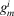, the X-ray linear absorption constant is given by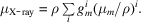In the case of neutrons used for powder diffraction, the absorption cross section is typically tabulated in barns (1 barn = 10−24 cm2); see Table 4.4.4.1 in International Tables for Crystallography, Volume C or websites such as http://www.ncnr.nist.gov/resources/n-lengths/list.html . The neutron absorption cross section is generally inversely proportional to velocity, and values are usually tabulated for neutrons with a speed of 2200 m s−1 (i.e., 25.3 meV kinetic energy, 1.80 Å wavelength). For neutrons, the equivalent expression with absorption cross sections depends on the number densities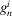(atoms/volume) of each element: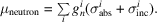Considering absorption, the effective volume is given by an integral over the sample,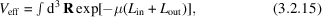where Lin and Lout are the paths of the incident and diffracted radiation to the point R within the sample, respectively.

The simplest case is of a sample in the form of a flat plate, with a thickness significantly greater than 1/μ, in the dividing position so that the angles of incidence and diffraction from the plane of the sample are both equal to θ. Let the dimensions of the beam be W in the equatorial direction (in the diffraction plane) and H in the axial direction (parallel to the diffractometer axis, i.e., perpendicular to the scattering plane) as shown in Fig. 3.2.2. Assume that the detector is large enough in the equatorial direction that all diffracted radiation is captured (i.e., no loss of signal due to parallax). Radiation scattered from a volume element a distance z below the surface of the sample will have a total path length 2z/sin θ; integration yields an effective sample volume of WH/2μ, independent of diffraction angle. The situation is more complicated if a flat sample is not optically deep or if the sample is shorter than W/sin θ; in either case, computation of the effective volume is straightforward, as long as the sample geometry is known with sufficient accuracy. The calculations are described in detail in Chapter 5.4of this volume.Figure 3.2.2 | top | pdf |Sketch illustrating symmetrical diffraction from a flat sample.

Another important case is that of a cylindrical sample, such as a Lindemann capillary for X-rays or a can of appropriate material (often vanadium) for neutrons. The integration for Veff above is given in convenient form for computation by Ida (2010), and is tabulated in International Tables for Crystallography, Volume C, Section 6.3.3.2. In the case of monochromatic radiation (neutrons or X-rays), for a given absorption constant μ, Veff is an increasing function of diffraction angle 2θ so that higher-angle peaks will appear relatively stronger. Weak-to-moderate absorption from a cylindrical sample affects the observed intensities in essentially the same algebraic form as a negative effective Debye–Waller factor (Hewat, 1979), leading to significantly underestimated (perhaps even negative) measured Debye–Waller factors. In the case of pulsed neutrons, the effect is compounded by the fact that the absorption cross section is generally inversely proportional to the neutron velocity; consequently, in a given detector, faster neutrons, representing larger |G|, will suffer less attenuation in the sample.

3.2.3.2. Absorption with multiphase samples

| top | pdf |

One important application of powder diffraction is the quantitative analysis of mixtures. Quantitative phase analysis is covered in detail in Chapter 3.9of this volume; here we set the stage. If a sample is a mixture of several phases, the powder-diffraction pattern will be the sum of the patterns of each phase, weighted by the fraction of the illuminated volume occupied by that phase. The most common configuration for quantitative analysis by X-ray diffraction is reflection from an optically deep flat-plate sample. In this case, the integrated intensity of a particular reflection from the jth phase is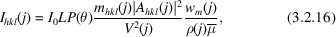where V(j), wm(j) and ρ(j) are the unit-cell volumes, mass fraction and density of phase j, respectively. Here I0 is an overall intensity scale factor, LP(θ) is the Lorentz and polarization factor common to all phases, and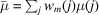is the absorption coefficient of all solid phases in the sample. The summation includes all solid phases, even amorphous materials.

Note that the intensity of peaks from a particular phase is not simply proportional to the fraction of that phase present. For example, if there are two phases A and B, the intensity of A peaks will be proportional to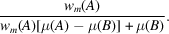3.2.3.3. Granularity, microabsorption and surface roughness

| top | pdf |

The above discussion of absorption in a powder sample is premised on the assumption that the powder sample is sufficiently fine that the beam traverses a large number of grains, i.e., that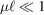, where μ is the absorption coefficient in the solid material andis a characteristic linear grain dimension. In that case, the effective linear absorption constant is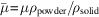, independent of the path that the radiation follows through the sample. However, if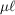is not very small, the simple picture of an average absorption length in the sample breaks down, leading to changes in the diffracted intensity.

There have been several treatments of microabsorption due to porosity and surface roughness. Rather than reviewing the literature, this section summarizes work of Hermann & Ermrich (1987) on a flat sample in the symmetrical reflection geometry discussed above. The single-phase sample is taken to be a collection of random polyhedra of average chord lengthwith packing fraction α0. The surface has the same lateral correlation length, but the average packing fraction rises from zero at the surface with characteristic dimension ts, i.e., α(z) = α0[1 − exp(−z/ts)], where z is the distance from the surface into the sample. ts = 0 represents an abrupt termination of the bulk porous material.

Hermann & Ermrich find that the diffracted intensity is reduced from the fine powder limit by a factor of 1 − PbulkPsurface, where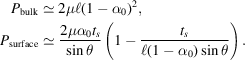If surface roughness is small, there is no dependence on diffraction angle 2θ, and the only effect is an overall reduction of diffracted intensity. Excess surface roughness (ts > 0), as might be produced by allowing a granular powder to settle against a flat surface, leads to additional diminution of diffracted intensity at low angles. The expression for Psurface given above is to leading order in 1/sin θ, and is therefore not valid for small grazing angles of incidence. Note that both the surface and bulk correction factors approach zero as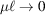. This analysis is in general agreement with analysis and measurements performed by Suortti (1972).

An additional effect of microabsorption in multi-component powders has been considered by Brindley (1945). The principle is illustrated in Fig. 3.2.3. An X-ray propagating through the sample (depicted by the broken line) suffers absorption according to the weighted average, but an X-ray diffracted in a grain of phase a has travelled further in that phase than average (solid line). If its path in the diffracting grain is x and the total path through the sample is L, absorption will reduce its intensity by a factor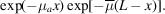This leads to a weaker observed reflection from a phase a with larger absorption constant μa if the particle size is an appreciable fraction of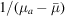.Figure 3.2.3 | top | pdf |Sketch illustrating an X-ray propagating through a mixture of two kinds of particles.

Averaging over the particle volume, we see that the observed intensity of phase a will be lowered from its value in a fine powder by a factor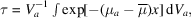where the integral is taken over one representative grain of phase a. This is exactly the integral of equation (3.2.15), and if one assumes monodisperse spherical particles, it is tabulated in International Tables for Crystallography, Volume C, Section 6.3.3.2and elsewhere, withreplacing μ. One hitch is that the tabulations are for μ > 0, whereas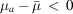for the less absorbing phases in a mixture. Brindley handles this with a series expansion and provides a table of τ versus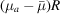.

By way of illustration, consider a mixture of equal weight fractions of corundum, magnetite and zircon powders, analysed with Cu Kα radiation. Fig. 3.2.4shows the weight fractions that would be measured from such a sample as a function of particle radius (assumed equal for all three phases). In this case, microabsorption biases the result by about 10% for particles of 10 µm diameter, and misses by a factor of two if the diameter is 70 µm.Figure 3.2.4 | top | pdf |Weight fractions that would be measured from a mixture of equal parts of three phases with Cu Kα radiation.

In considering microabsorption, there are several important points to note. This entire analysis is only valid for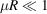, since otherwise the concept of an average absorption length becomes ill defined. That means that the Brindley analysis breaks down precisely when it becomes significant. Of course, the Brindley assumption of monodisperse spherical particles is unrealistic for most real samples. Both the surface roughness and Brindley corrections depend on diffraction angle as well as μR, but, as noted above, for moderate absorption, the angle dependence appears as an effective Debye–Waller factor. The parameter R is the radius of the grain of material, which is commonly very much larger than the size of a coherently diffracting region; a Scherrer analysis of particle size through line broadening does not provide a useful estimate of R. Most importantly, unless one has an accurate independent measurement of the size and shape of the grains, microabsorption corrections should be taken as a sign of impending trouble, not a quantitative correction.

| top | pdf |

Strain broadening is often observed not to be monotonically dependent on the magnitude of the d-spacing, but to have a more complicated dependence on the direction of the diffraction vector G. An illustration of the effect in cubic Rb3C60 is shown in Fig. 3.2.5(Stephens, 1999). The lower part of the figure shows an apparently irregular trend of diffraction peak widths on diffraction angle 2θ.Figure 3.2.5 | top | pdf |Diffraction peak width versus diffraction angle from a powder pattern of face-centred cubic Rb3C60 (Stephens, 1999). The inset at the top shows a limited range of the data, fitted by a model with two different peak widths (upper trace) and with the widths constrained to be equal (lower trace).

Whatever the nature of random internal stresses and strains, it can be argued on general grounds that strain broadening depends on a combination of fourth-rank tensors. On the assumption that the distribution of random strains is Gaussian, each diffraction peak has Gaussian shape, and the variance of the inverse d-spacing squared is a quartic form in the reflection indices (hkl). This can be expressed as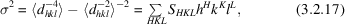where the sum is over terms with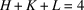. This leads to a contribution to the width of a diffraction peak proportional to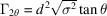for angle-dispersive measurements, and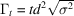for time-of-flight neutron measurements. Strain broadening in real samples frequently leads to peak shapes that are closer to Lorentzian than Gaussian, and the justification of this expression breaks down because the second moment of the distribution of d−2 is then not defined. Nevertheless, this method is often successful at modelling the phenomenology of anisotropic peak broadening.

The parameters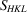arise from the nature of the strain and the values of elastic constants for any particular sample. Typically, they must therefore be regarded as phenomenological, and can be adjusted in fitting a model to observed data. They are constrained by the symmetry of the Laue group of the sample, which leads to restrictions on theterms for the various Laue classes, listed in Table 3.2.1(Popa, 1998).

 Table 3.2.1| top | pdf | Restrictions and reflections of anisotropic strain parameters for the various Laue classes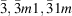: hexagonal indices.
Class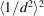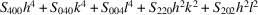+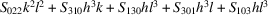+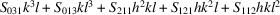2/m (b-axis unique)+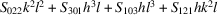2/mmm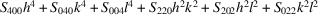4/m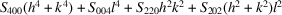+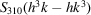4/mmm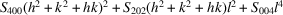+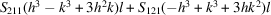+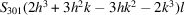+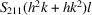HexagonalCubic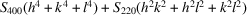3.2.3.5. Preferred orientation (texture)

| top | pdf |

The discussion of diffraction-peak intensities in Section 3.2.2.2above presumes that the sample is a random powder, with every orientation equally probable. Real samples frequently exhibit some deviation from that ideal case, perhaps because of the way that grains of platy or acicular (needle-like) habit settle into a sample holder, or because a solid polycrystalline sample crystallized or grew anisotropically, or was formed or worked as a solid. The latter case is generally called texture, and can provide a fruitful basis for understanding the history or mechanical properties of a sample. The topic is further discussed in Chapter 5.3of this volume. For the present purposes, deviation from a collection of randomly oriented grains will be regarded as an artifact to be minimized and/or modelled.

The distribution of orientations of crystallites in a sample can be studied using pole figures. For a given reflection G, the pole figure is defined as the probability density over a spherical surface that G falls in a particular direction relative to the sample. For a random powder, all pole figures would be uniform; for a single crystal, each pole figure would be a set of delta functions. Modelling of preferred orientation in the sample is accomplished by multiplying the theoretical intensity by the pole-figure density in the direction of the diffraction vector. Here we discuss two different treatments of preferred orientation.

The symmetrized spherical-harmonic approach follows Järvinen (1993), considering samples that are axially symmetric about some axis P. Experimentally, this can be ensured by rotating the sample about P during data collection. Take α to be the angle between P and the diffraction vector: α is zero for a conventional symmetrical flat plate and 90° for Debye–Scherrer geometry.

For a given reflection hkl, the polar-axis density in the diffraction direction may be expanded in spherical harmonics as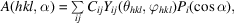where Yij and Pi are the usual spherical harmonics and Legendre polynomials, and θhkl andare the spherical coordinates of the hkl reflection relative to some chosen axis of the crystal.

In general, there will be several reflections equivalent to hkl by the Laue symmetry of the crystalline phase, and so symmetry-adapted functions should replace the spherical harmonics in the above equation. Only even orders i are considered, because of the inversion symmetry of diffraction (neglecting imaginary atomic scattering amplitudes). The Laue symmetry of the crystalline phase implies that certain of the harmonics are zero; see the original article by Järvinen (1993) for explicit functional forms of the harmonics and a tabulation of which are allowed for each Laue group.

The second approach considered here is the widely used March (1932)–Dollase (1986) model, which is applicable to the most common powder-diffraction sample geometries of axial symmetry either along the diffraction vector or perpendicular to the diffraction plane, for samples whose preferred orientation arises from the settling of either disc- or rod-shaped crystallites in the sample holder. The cylinder axis H governs the preferred orientation. For any Bragg reflection G, the pole density in the diffraction direction is hypothesized to be a particular function of the angle α between H and G, viz.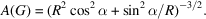Here R is a parameter describing the degree of preferred orientation; it is less than unity for maximum pole density at α = 0, i.e.,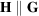, as would be found for disc-like samples preferentially lying in the reflection plane. R > 1 corresponds to maximum pole density at α = 90°, as might be found for acicular crystallites in either a flat-plate or Debye–Scherrer geometry. The particular functional form has some theoretical justification as a model for grain rotation in settling of a granular sample, as well as being properly normalized, suitable for oblate or prolate grains with a single parameter, and in agreement with pole distributions observed in many samples. To apply this model to powder-diffraction data, one must find the appropriate axis H, either through prior knowledge of growth habit or by trial and error.

3.2.3.6. Extinction

| top | pdf |

The analysis of diffracted intensities in this chapter has been premised on the assumption that the diffracted beam produced by each crystallite is much weaker than the incident beam, known as the kinematic approximation. This is a consequence of the Born approximation, whereby the diffracted intensity from one grain is proportional to the number of atoms within it. However, as the size of the crystallite grows, so will the intensity of the diffracted wave within it. The diffracted radiation will be re-diffracted back into the incident beam, leading to a measured integrated intensity less than the kinematic value, by a ratio y(G) = Iobs(G)/Ikinematic(G), which depends on the particular Bragg reflection G. This is the phenomenon of primary extinction, which can be understood within the framework of the dynamical theory of diffraction. The following discussion ignores absorption.

The relevant physical parameter is the extinction length Λ. For X-rays,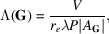where V is the unit-cell volume, re = 2.82 × 10−15 m, AG is the structure factor, and P is the polarization factor: 1 or cos2 2θ for S or P polarization, respectively. For neutrons,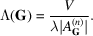As an example, the 111 reflection of Si has an extinction length of 7.2 µm for 1.54 Å X-rays and 50 µm for 1.59 Å neutrons. Intensities from the kinematic theory are correct in the limit that the coherent grain size is much less than Λ. Note that extinction is most significant for the strongest reflections in a powder-diffraction pattern.

There are no exact calculations of extinction available for sample geometries applicable to powder samples, but Thorkildsen and Larsen have obtained a rigorous series solution for spherical particles of radius R in powers of Λ/R (Thorkildsen & Larsen, 1998). To lowest order,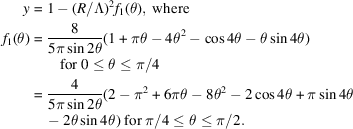This first term gives y accurate to 3% for R/Λ ≤ 0.4. Fig. 3.2.6shows their calculated result up to fifth order.Figure 3.2.6 | top | pdf |Extinction correction for spherical particles according to Thorkildsen & Larsen (1998).

References

Brindley, G. W. (1945). The effect of grain or particle size on x-ray reflections from mixed powders and alloys, considered in relation to the quantitative determination of crystalline substances by x-ray methods. Philos. Mag. 36, 347–369.Google Scholar
Dollase, W. A. (1986). Correction of intensities for preferred orientation in powder diffractometry: application of the March model. J. Appl. Cryst. 19, 267–272.Google Scholar
Hermann, H. & Ermrich, M. (1987). Microabsorption of X-ray intensity in randomly packed powder specimens. Acta Cryst. A43, 401–405.Google Scholar
Hewat, A. W. (1979). Absorption corrections for neutron diffraction. Acta Cryst. A35, 248.Google Scholar
Ida, T. (2010). Efficiency in the calculation of absorption corrections for cylinders. J. Appl. Cryst. 43, 1124–1125.Google Scholar
Järvinen, M. (1993). Application of symmetrized harmonics expansion to correction of the preferred orientation effect. J. Appl. Cryst. 26, 525–531.Google Scholar
March, A. (1932). Mathematische Theorie der Regelung nach der Korngestah bei affiner Deformation. Z. Kristallogr. 81, 285–297.Google Scholar
Popa, N. C. (1998). The (hkl) dependence of diffraction-line broadening caused by strain and size for all Laue groups in Rietveld refinement. J. Appl. Cryst. 31, 176–180.Google Scholar
Stephens, P. W. (1999). Phenomenological model of anisotropic peak broadening in powder diffraction. J. Appl. Cryst. 32, 281–289.Google Scholar
Suortti, P. (1972). Effects of porosity and surface roughness on the X-ray intensity reflected from a powder specimen. J. Appl. Cryst. 5, 325–331.Google Scholar
Thorkildsen, G. & Larsen, H. B. (1998). Primary extinction in cylinders and spheres. Acta Cryst. A54, 172–185.Google Scholar